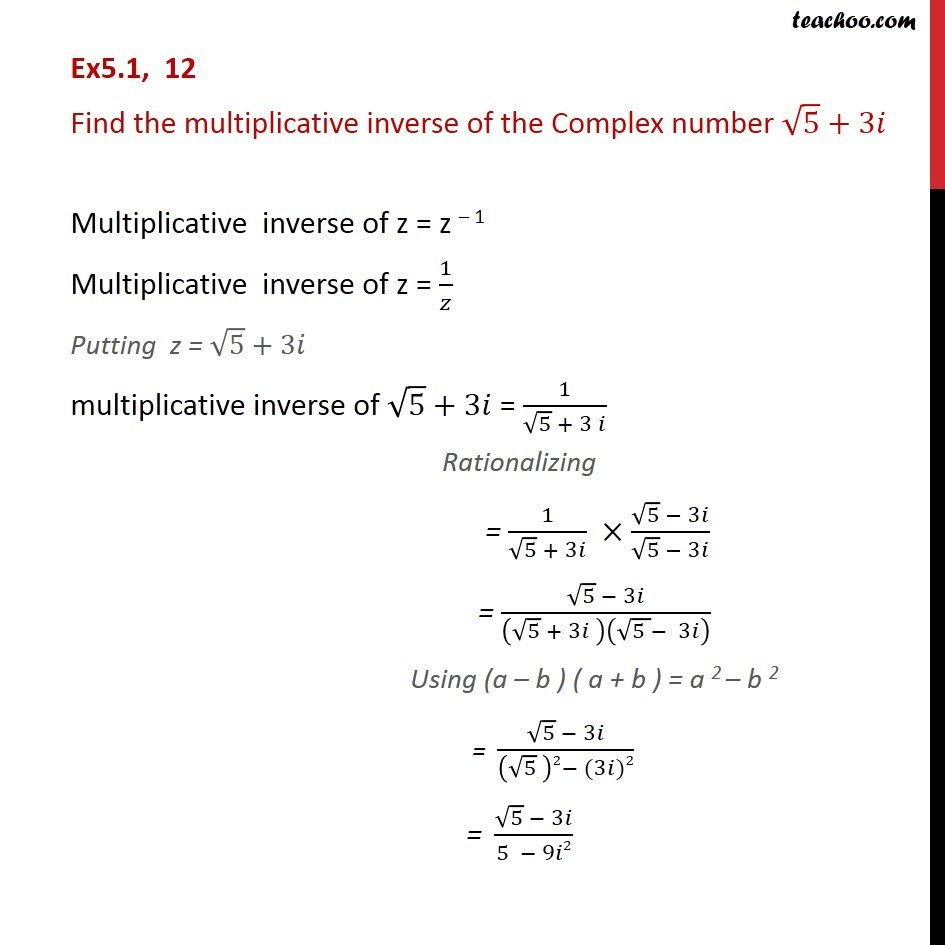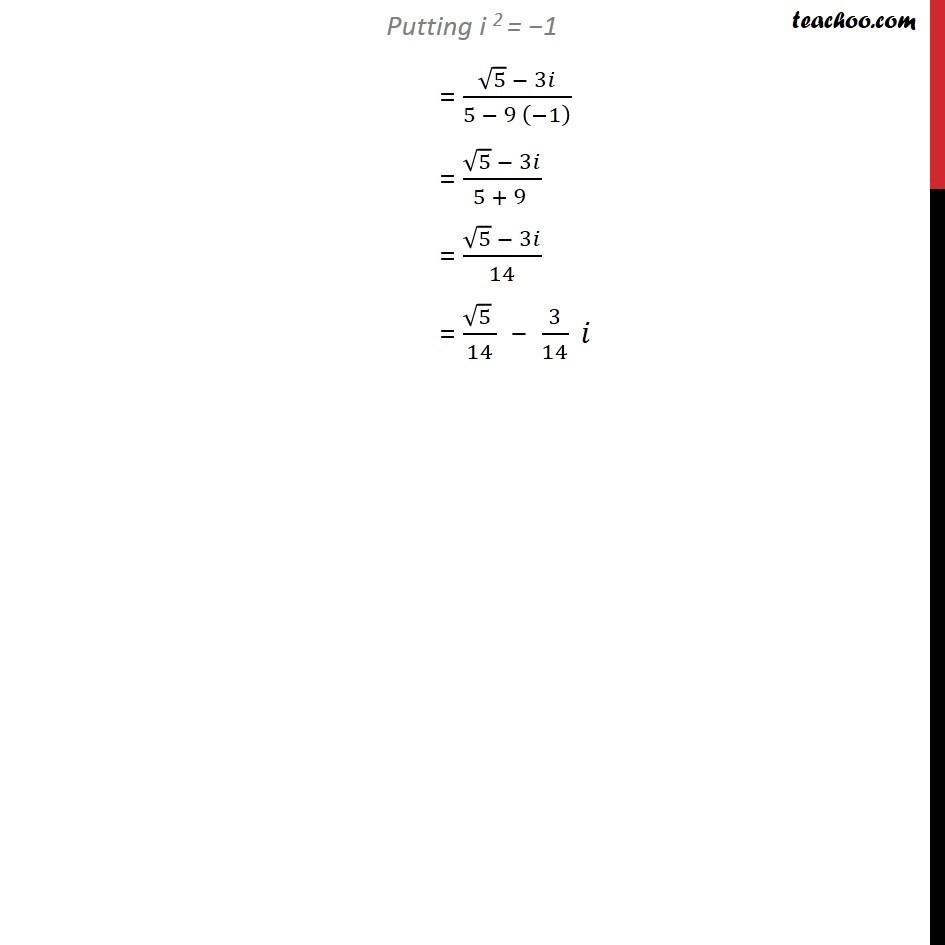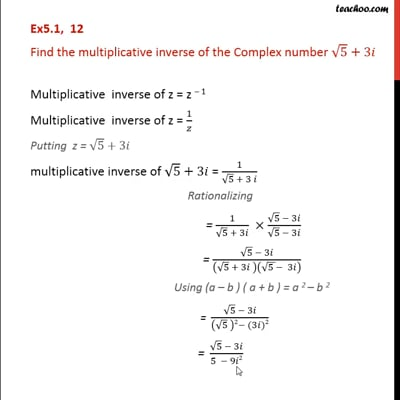Ex 5.1

Chapter 5 Class 11 Complex Numbers (Term 1)
Serial order wiseThis video is only available for Teachoo black users

### Transcript

Ex5.1, 12 Find the multiplicative inverse of the Complex number √5 + 3𝑖 Multiplicative inverse of z = z – 1 Multiplicative inverse of z = 1/𝑧 Putting z = √5 + 3𝑖 multiplicative inverse of √5 + 3𝑖 = 1/(√5 + 3 𝑖) Rationalizing = 1/(√5 + 3𝑖) ×(√5 − 3𝑖)/(√5 − 3𝑖) = (√5 − 3𝑖)/(√5 + 3𝑖 )(√(5 )− 3𝑖) Using (a – b ) ( a + b ) = a 2 – b 2 = (√5 − 3𝑖)/((√5 )2− (3𝑖)2) "= " (√5 − 3𝑖)/(5 − 9𝑖2) Putting i 2 = −1 = (√5 − 3𝑖)/(5 − 9 (−1) ) = (√5 − 3𝑖)/(5 + 9 ) = (√5 − 3𝑖)/14 = (√5 )/14 − 3/14 𝑖